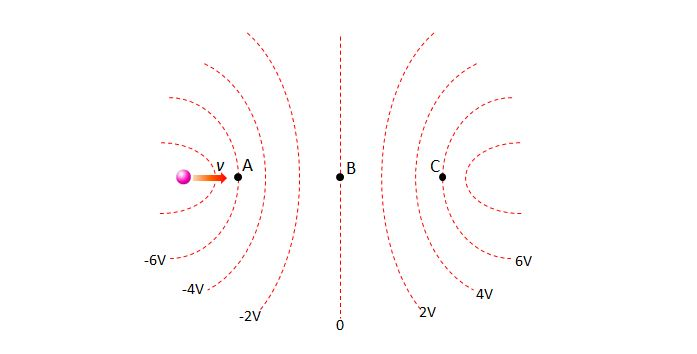# Motion of charged particle in electric fieldIn the above diagram, a charged particle is moving in an electric field, where the dotted red lines are equipotential lines. The charged particle is initially entered with a speed of $v$ from the left of point A. Then it passes points A and B, finally arriving at point C. It stops for a moment there at point C, and then starts to move back to point A. Which of the following statements is correct?

a) The charged particle is negatively charged.
b) The magnitude of the kinetic energy when the charged particle passes point B is half the kinetic energy when the charged particle passes point A.
c) The force acting on the charged particle at point C is not zero.

×